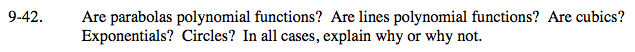Home > A2C > Chapter 9 > Lesson 9.1.2 > Problem9-42

9-42.

Are parabolas polynomial functions? Are lines polynomial functions? Are cubics? Exponentials? Circles? In all cases, explain why or why not. Homework Help ✎Try writing an equation for each type of graph.

Parabolas, lines, and cubics are all polynomial functions. Exponentials and circles are not.